Scalar product - Defination

Chapter 10 Class 12 Vector Algebra
Concept wise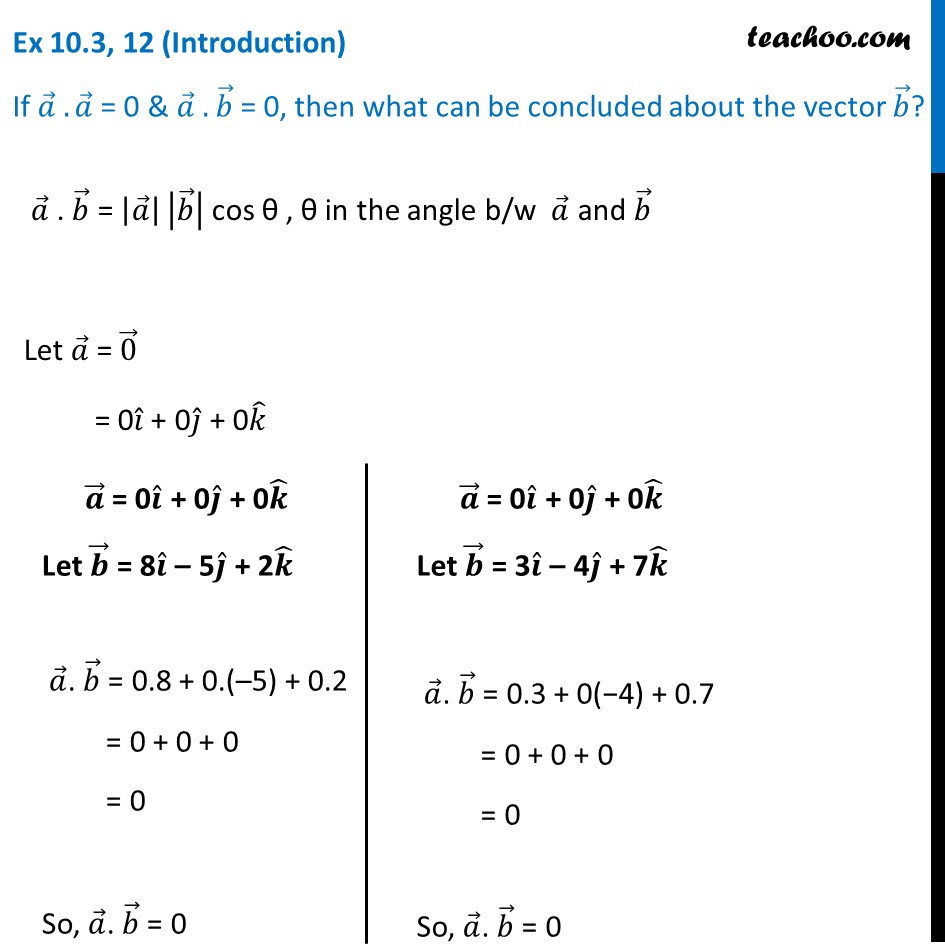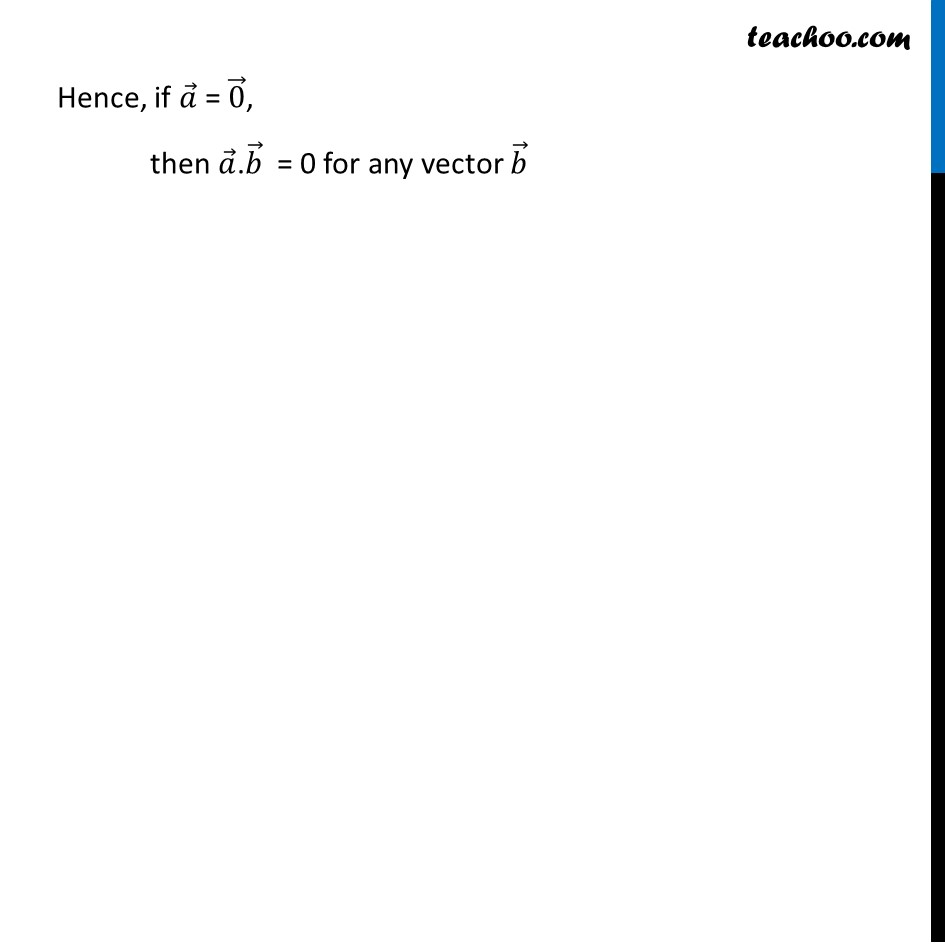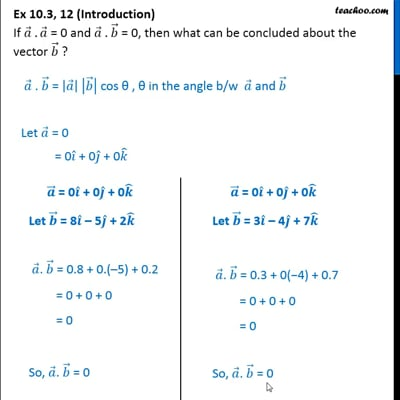This video is only available for Teachoo black users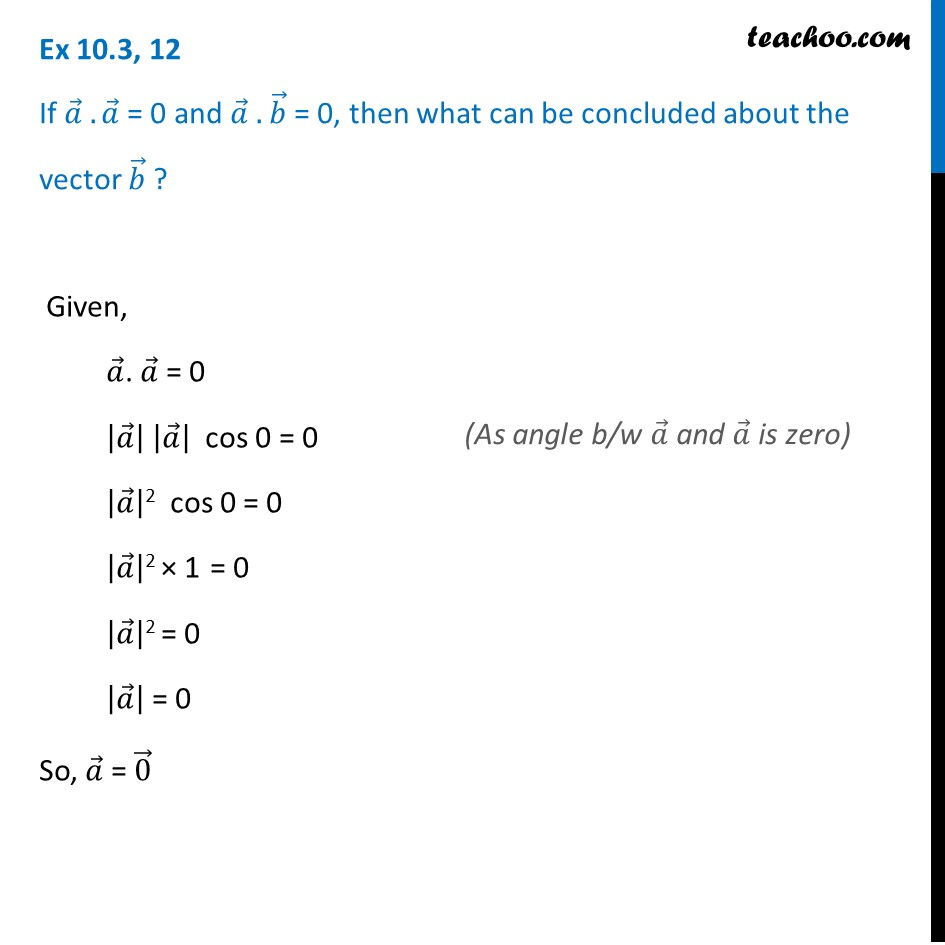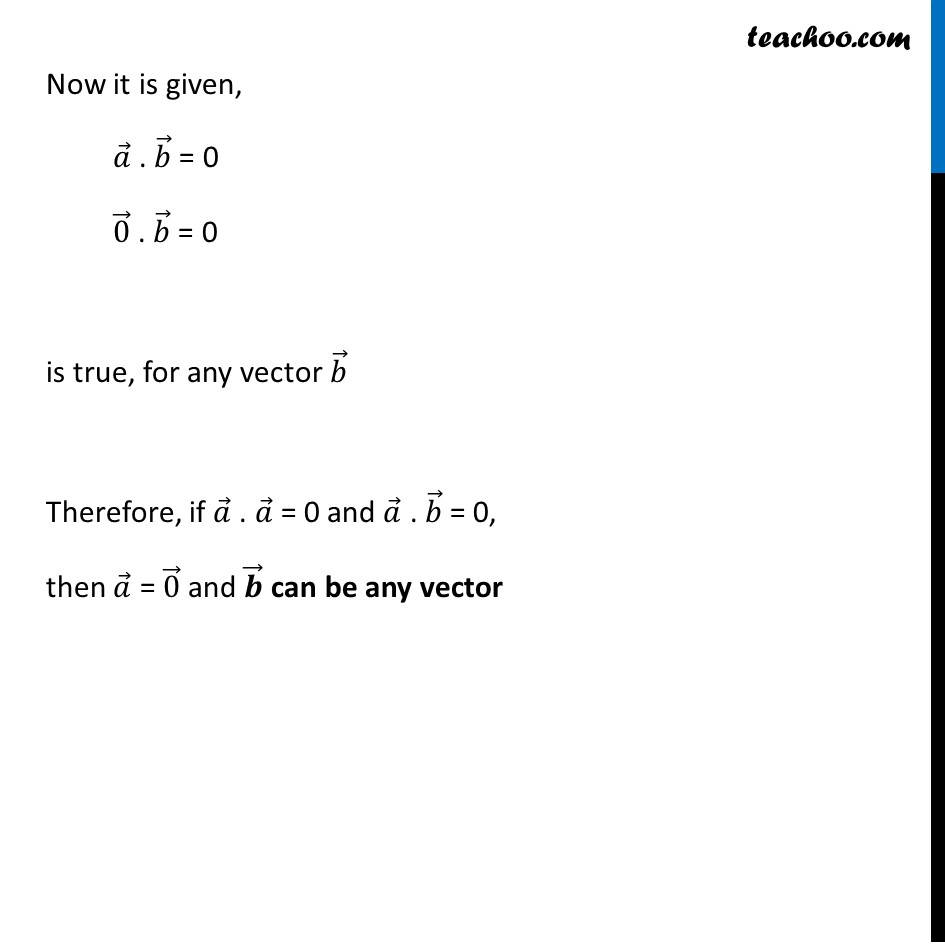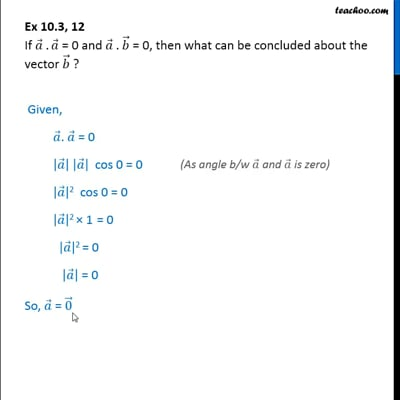This video is only available for Teachoo black users

Introducing your new favourite teacher - Teachoo Black, at only ₹83 per month

### Transcript

Ex 10.3, 12 (Introduction) If 𝑎 ⃗ .𝑎 ⃗ = 0 & 𝑎 ⃗ . 𝑏 ⃗ = 0, then what can be concluded about the vector 𝑏 ⃗? 𝑎 ⃗ . 𝑏 ⃗ = |𝑎 ⃗ | |𝑏 ⃗ | cos θ , θ in the angle b/w 𝑎 ⃗ and 𝑏 ⃗ Let 𝑎 ⃗ = 0 ⃗ = 0𝑖 ̂ + 0𝑗 ̂ + 0𝑘 ̂ 𝒂 ⃗ = 0𝒊 ̂ + 0𝒋 ̂ + 0𝒌 ̂ Let 𝒃 ⃗ = 8𝒊 ̂ – 5𝒋 ̂ + 2𝒌 ̂ 𝑎 ⃗. 𝑏 ⃗ = 0.8 + 0.(–5) + 0.2 = 0 + 0 + 0 = 0 So, 𝑎 ⃗. 𝑏 ⃗ = 0 𝒂 ⃗ = 0𝒊 ̂ + 0𝒋 ̂ + 0𝒌 ̂ Let 𝒃 ⃗ = 3𝒊 ̂ – 4𝒋 ̂ + 7𝒌 ̂ 𝑎 ⃗. 𝑏 ⃗ = 0.3 + 0(−4) + 0.7 = 0 + 0 + 0 = 0 So, 𝑎 ⃗. 𝑏 ⃗ = 0 Hence, if 𝑎 ⃗ = 0 ⃗, then 𝑎 ⃗.𝑏 ⃗ = 0 for any vector 𝑏 ⃗ Ex 10.3, 12 If 𝑎 ⃗ .𝑎 ⃗ = 0 and 𝑎 ⃗ . 𝑏 ⃗ = 0, then what can be concluded about the vector 𝑏 ⃗ ? Given, 𝑎 ⃗. 𝑎 ⃗ = 0 |𝑎 ⃗ | |𝑎 ⃗ | cos 0 = 0 |𝑎 ⃗ |2 cos 0 = 0 |𝑎 ⃗ |2 × 1 = 0 |𝑎 ⃗ |2 = 0 |𝑎 ⃗ | = 0 So, 𝑎 ⃗ = 0 ⃗ Now it is given, 𝑎 ⃗ . 𝑏 ⃗ = 0 0 ⃗ . 𝑏 ⃗ = 0 is true, for any vector 𝑏 ⃗ Therefore, if 𝑎 ⃗ . 𝑎 ⃗ = 0 and 𝑎 ⃗ . 𝑏 ⃗ = 0, then 𝑎 ⃗ = 0 ⃗ and 𝒃 ⃗ can be any vector